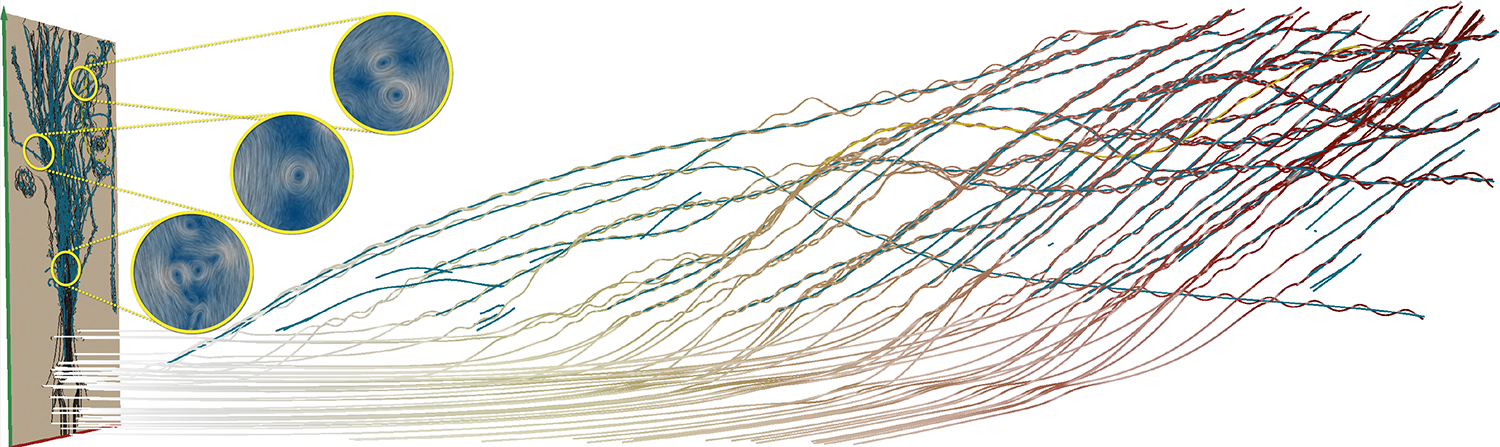## Vortex Lens: Interactive Vortex Core Line Extraction using Observed Line Integral Convolution

#### Peter Rautek, Xingdi Zhang, Bernhard Woschizka, Thomas Theußl and Markus Hadwiger##### IEEE Transactions on Visualization and Computer Graphics, Vol.30, No.1 (Proceedings IEEE VIS 2023), to appear , 2024

This paper describes a novel method for detecting and visualizing vortex structures in unsteady 2D fluid flows. The method is based on an interactive local reference frame estimation that minimizes the observed time derivative of the input flow field V(x,t). A locally optimal reference frame W(x,t) assists the user in the identification of physically observable vortex structures in Observed Line Integral Convolution (LIC) visualizations. The observed LIC visualizations are interactively computed and displayed in a user-steered vortex lens region, embedded in the context of a conventional LIC visualization outside the lens. The locally optimal reference frame is then used to detect observed critical points, where V = W, which are used to seed vortex core lines. Each vortex core line is computed as a solution of the ordinary differential equation (ODE) w'(t) = W(w(t),t), with an observed critical point as initial condition (w(t0),t0). During integration, we enforce a strict error bound on the difference between the extracted core line and the integration of a path line of the input vector field, i.e., a solution to the ODE v'(t) = V(v(t),t). We experimentally verify that this error depends on the step size of the core line integration. This ensures that our method extracts Lagrangian vortex core lines that are the simultaneous solution of both ODEs with a numerical error that is controllable by the integration step size. We show the usability of our method in the context of an interactive system using a lens metaphor, and evaluate the results in comparison to state-of-the-art vortex core line extraction methods.

@article{Rautek2023VortexLens,
title = {Vortex Lens: Interactive Vortex Core Line Extraction using Observed Line Integral Convolution},
author = {Rautek, Peter and Zhang, Xingdi and Woschizka, Bernhard and Theu{\ss}l, Thomas and Hadwiger, Markus},
journal = {IEEE Transactions on Visualization and Computer Graphics (Proceedings IEEE VIS 2023)},
year = {2024},
volume = {30},
number = {1},
pages = {to appear}
}